CUET  >  Analytical Reasoning Quiz II, Non Verbal Reasoning

# Analytical Reasoning Quiz II, Non Verbal Reasoning - CUET

Test Description

## 15 Questions MCQ Test General Test Preparation for CUET - Analytical Reasoning Quiz II, Non Verbal Reasoning

Analytical Reasoning Quiz II, Non Verbal Reasoning for CUET 2023 is part of General Test Preparation for CUET preparation. The Analytical Reasoning Quiz II, Non Verbal Reasoning questions and answers have been prepared according to the CUET exam syllabus.The Analytical Reasoning Quiz II, Non Verbal Reasoning MCQs are made for CUET 2023 Exam. Find important definitions, questions, notes, meanings, examples, exercises, MCQs and online tests for Analytical Reasoning Quiz II, Non Verbal Reasoning below.
Solutions of Analytical Reasoning Quiz II, Non Verbal Reasoning questions in English are available as part of our General Test Preparation for CUET for CUET & Analytical Reasoning Quiz II, Non Verbal Reasoning solutions in Hindi for General Test Preparation for CUET course. Download more important topics, notes, lectures and mock test series for CUET Exam by signing up for free. Attempt Analytical Reasoning Quiz II, Non Verbal Reasoning | 15 questions in 30 minutes | Mock test for CUET preparation | Free important questions MCQ to study General Test Preparation for CUET for CUET Exam | Download free PDF with solutions
 1 Crore+ students have signed up on EduRev. Have you?
Analytical Reasoning Quiz II, Non Verbal Reasoning - Question 1

### Find the number of triangles in the given figure.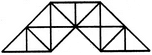Detailed Solution for Analytical Reasoning Quiz II, Non Verbal Reasoning - Question 1

The figure may be labelled as shown.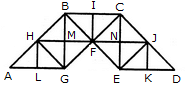The simplest triangles are AHL, LHG, GHM, HMB, GMF, BMF, BIF, CIF, FNC, CNJ, FNE, NEJ, EKJ and JKD i.e. 14 in number.

The triangles composed of two components each are AGH, BHG, HBF, BFG, HFG, BCF, CJF, CJE, JEF, CFE and JED i.e. 11 in number.

The triangles composed of four components each are ABG, CBG, BCE and CED i.e. 4 in number.

Total number of triangles in the given figure = 14 + 11 + 4 = 29.

Analytical Reasoning Quiz II, Non Verbal Reasoning - Question 2

### Find the number of triangles in the given figure.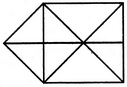Detailed Solution for Analytical Reasoning Quiz II, Non Verbal Reasoning - Question 2

The figure may be labelled as shown.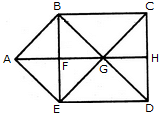The simplest triangles are ABF, BFG, BCG, CGH, GHD, GED, EFG and AFE i.e. 8 in number.

The triangles composed of two components each are ABG, BGE, AGE, ABE and GCD i.e. 5 in number.

The triangles composed of three components each are BCD, CDE, BED and BCE i.e. 4 in number.

Thus, there are 8 + 5 + 4 = 17 triangles in the figure.

Analytical Reasoning Quiz II, Non Verbal Reasoning - Question 3

### Find the number of triangles in the given figure.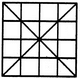Detailed Solution for Analytical Reasoning Quiz II, Non Verbal Reasoning - Question 3

The figure may be labelled as shown.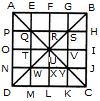The simplest triangles are APQ, AEQ, QTU, QRU, BGS, BHS, RSU, SUV, TUW, UWX, NWD, WDM, UVY, UXY, JCY and YKC i.e. 16 in number.

The triangles composed of two components each are QUW, QSU, SYU and UWY i.e. 4 in number.

The triangles composed of three components each are AOU, AFU, FBU, BIU, UIC, ULC, ULD and OUD i.e. 8 in number.

The triangles composed of four components each are QYW, QSW, QSY and SYW i.e. 4 in number.

The triangles composed of six components each are AUD, ABU, BUC and DUC i.e. 4 in number.

The triangles composed of seven components each are QMC, ANY, EBW, PSD, CQH, AGY, DSK and BJW i.e. 8 in number.

The triangles composed of twelve components each are ABD, ABC, BCD and ACD i.e. 4 in number.

Thus, there are 16 + 4 + 8 + 4 + 4 + 8 + 4 = 48 triangles in the figure.

Analytical Reasoning Quiz II, Non Verbal Reasoning - Question 4

Find the number of triangles in the given figure.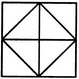Detailed Solution for Analytical Reasoning Quiz II, Non Verbal Reasoning - Question 4

The figure may be labelled as shown.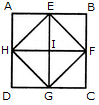The simplest triangles are AEH, EHI, EBF, EFI, FGC, IFG, DGH and HIG i.e. 8 in number.

The triangles composed of two components each are HEF, EFG, HFG and EFG i.e. 4 in number.

Thus, there are 8 + 4 = 12 triangles in the figure.

Analytical Reasoning Quiz II, Non Verbal Reasoning - Question 5

Find the number of triangles in the given figure.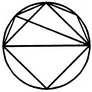Detailed Solution for Analytical Reasoning Quiz II, Non Verbal Reasoning - Question 5

The figure may be labelled as shown.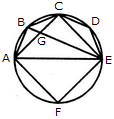The simplest triangles are ABG, BCG, CGE, CDE, AGE and AEF i.e. 6 in number.

The triangles composed of two components each are ABE, ABC, BCE and ACE i.e. 4 in number.

There are 6 + 4 = 10 triangles in the figure.

Analytical Reasoning Quiz II, Non Verbal Reasoning - Question 6

Find the number of triangles in the given figure.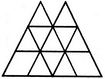Detailed Solution for Analytical Reasoning Quiz II, Non Verbal Reasoning - Question 6

The figure may be labelled as shown.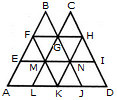The simplest triangles are BFG, CGH, EFM, FMG, GMN, GHN, HNI, LMK, MNK and KNJ i.e. 10 in number.

The triangles composed of three components each are FAK and HKD i.e. 2 in number.

The triangles composed of four components each are BEN, CMI, GLJ and FHK i.e. 4 in number.

The triangles composed of eight components each are BAJ and OLD i.e. 2 in number.

Thus, there are 10 + 2 + 4 + 2 = 18 triangles in the given figure.

Analytical Reasoning Quiz II, Non Verbal Reasoning - Question 7

Find the number of triangles in the given figure.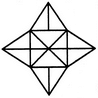Detailed Solution for Analytical Reasoning Quiz II, Non Verbal Reasoning - Question 7

The figure may be labelled as shown.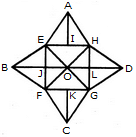The simplest triangles are AEI, AIH, BEJ, BJF, CFK, CKG, DGL, DLH, EOJ, FOJ, FOG, LOG, HOL and HOE i.e. 14 in number.

The triangles composed of two components each are EAH, FBE, BEO, EOF, BFO, FCG, GDH, HOD, HOG and GOD i.e. 10 in number.

The triangles composed of three components each are EFH, EHG, FGH and EFG i.e. 4 in number.

Thus, there are 14 + 10 + 4 = 28 triangles in the given figure.

Analytical Reasoning Quiz II, Non Verbal Reasoning - Question 8

Find the number of triangles in the given figure.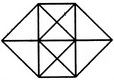Detailed Solution for Analytical Reasoning Quiz II, Non Verbal Reasoning - Question 8

The figure may be labelled as shown.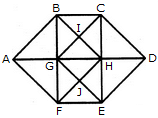The simplest triangles are ABG, BIG, BIC, CIH, GIH, CDH, HED, GHJ, HJE, FEJ, GFJ and AGF i.e. 12 in number.

The triangles composed of two components each are ABF, CDE, GBC, BCH, GHG, BHG, GHF, GHE, HEF and GEF i.e. 10 in number.

The triangles composed of three components each are ABH, AFH, CDG and GDE i.e. 4 in number.

The triangles composed of four components each are BHF and CGE i.e. 2 in number.

Total number of triangles in the figure = 12 + 10 + 4 + 2 = 28.

Analytical Reasoning Quiz II, Non Verbal Reasoning - Question 9

Find the minimum number of straight lines required to make the given figure.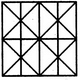Detailed Solution for Analytical Reasoning Quiz II, Non Verbal Reasoning - Question 9

The figure may be labelled as shown.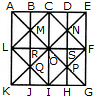The horizontal lines are AK, BJ, CI, DH and EG i.e. 5 in number.

The vertical lines are AE, LF and KG i.e. 3 in number.

The slanting lines are LC, CF, FI, LI, EK and AG i.e. 6 in number.

Thus, there are 5 + 3 + 6 = 14 straight lines in the figure.

Analytical Reasoning Quiz II, Non Verbal Reasoning - Question 10

What is the number of straight lines and the number of triangles in the given figure.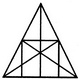Detailed Solution for Analytical Reasoning Quiz II, Non Verbal Reasoning - Question 10

The figure may be labelled as shown.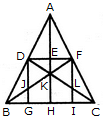The Horizontal lines are DF and BC i.e. 2 in number.

The Vertical lines are DG, AH and FI i.e. 3 in number.

The Slanting lines are AB, AC, BF and DC i.e. 4 in number.

Thus, there are 2 + 3 + 4 = 9 straight lines in the figure.

Now, we shall count the number of triangles in the figure.

The simplest triangles are ADE, AEF, DEK, EFK, DJK, FLK, DJB, FLC, BJG and LIC i.e. 10 in number.

The triangles composed of two components each are ADF, AFK, DFK, ADK, DKB, FCK, BKH, KHC, DGB and FIC i.e. 10 in number.

The triangles composed of three components each are DFJ and DFL i.e. 2 in number.

The triangles composed of four components each are ABK, ACK, BFI, CDG, DFB, DFC and BKC i.e. 7 in number.

The triangles composed of six components each are ABH, ACH, ABF, ACD, BFC and CDB i.e. 6 in number.

There is only one triangle i.e. ABC composed of twelve components.

There are 10 + 10 + 2 + 7 + 6+ 1 = 36 triangles in the figure.

Analytical Reasoning Quiz II, Non Verbal Reasoning - Question 11

Find the number of triangles in the given figure.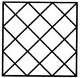Detailed Solution for Analytical Reasoning Quiz II, Non Verbal Reasoning - Question 11

The figure may be labelled as shown.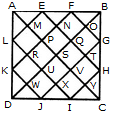The simplest triangles are AML, LRK, KWD, DWJ, JXI, IYC, CYH, HTG, GOB, BOF, FNE and EMA i.e. 12 in number.

The triangles composed of two components each are AEL, KDJ, HIC and FBG i.e. 4 in number.

The triangles composed of three components each are APF, EQB, BQH, GVC, CVJ, IUD, DUL and KPA i.e. 8 in number.

The triangles composed of six components each are ASB, BSC, CSD, DSA, AKF, EBH, CGJ and IDL i.e. 8 in number.

The triangles composed of twelve components each are ADB, ABC, BCD and CDA i.e. 4 in number.

Total number of triangles in the figure = 12 + 4 + 8 + 8 + 4 = 36.

Analytical Reasoning Quiz II, Non Verbal Reasoning - Question 12

What is the number of triangles that can be formed whose vertices are the vertices of an octagon but have only one side common with that of octagon?

Detailed Solution for Analytical Reasoning Quiz II, Non Verbal Reasoning - Question 12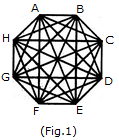When the triangles are drawn in an octagon with vertices same as those of the octagon and having one side common to that of the octagon, the figure will appear as shown in (Fig. 1).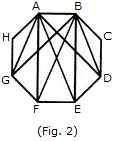Now, we shall first consider the triangles having only one side AB common with octagon ABCDEFGH and having vertices common with the octagon (See Fig. 2).Such triangles are ABD, ABE, ABF and ABG i.e. 4 in number.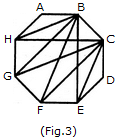Similarly, the triangles having only one side BC common with the octagon and also having vertices common with the octagon are BCE, BCF, BCG and BCH (as shown in Fig. 3). i.e. There are 4 such triangles.

This way, we have 4 triangles for each side of the octagon. Thus, there are 8 x 4 = 32 such triangles.

Analytical Reasoning Quiz II, Non Verbal Reasoning - Question 13

Find the number of triangles in the given figure.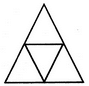Detailed Solution for Analytical Reasoning Quiz II, Non Verbal Reasoning - Question 13

The figure may be labelled as shown.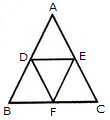The simplest triangles are ADE, BDF, DEF and EFC i.e. 4 in number.

There is only one triangle ABC composed of four components.

Thus, there are 4+1 = 5 triangles in the given figure.

Analytical Reasoning Quiz II, Non Verbal Reasoning - Question 14

Find the number of triangles in the given figure.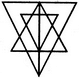Detailed Solution for Analytical Reasoning Quiz II, Non Verbal Reasoning - Question 14

The figure may be labelled as shown.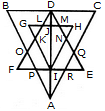The simplest triangles are GLK, DLJ, DJM, HMN, QRE, IRA, IPA and FPO i.e. 8 in number.

The triangles having two components each are BDO, CDQ, DLM, PRA, KFI, NEI, HJI, GJI, DKI and DNI i.e. 10 in number.

The triangles having four components each are DIE, DFI, DOA, DQA andGHI i.e. 5 in number.

The triangles having six components each are DCA and DBA i.e. 2 in number.

DEF is the only triangle having eight components.

ABC is the only triangle having twelve components.

Thus, there are 8+10+ 5 + 2+1 + 1 = 27 triangles in the figure.

Analytical Reasoning Quiz II, Non Verbal Reasoning - Question 15

Find the number of triangles in the given figure.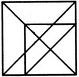Detailed Solution for Analytical Reasoning Quiz II, Non Verbal Reasoning - Question 15

The figure may be labelled as shown.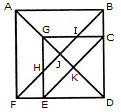The simplest triangles are EFH, BIC, GHJ, GIJ, EKD and CKD i.e. 6 in number.

The triangles composed of two components each are ABJ, AFJ, GCK, GEK, CED arid GHI i.e. 6 in number.

The triangles composed of three components each are GCD, GED, DJB and DJF i.e. 4 in number.

The triangles composed of four components each are ABF and GCE i.e. 2 in number.

The triangles composed of five components each are ABD and AFD i.e. 2 in number.

There is only one triangle i.e. FBD composed of six components.

Total number of triangles in the figure = 6 + 6 + 4 + 2 + 2 + 1 = 21.

## General Test Preparation for CUET

177 videos|324 docs|398 tests
Information about Analytical Reasoning Quiz II, Non Verbal Reasoning Page
In this test you can find the Exam questions for Analytical Reasoning Quiz II, Non Verbal Reasoning solved & explained in the simplest way possible. Besides giving Questions and answers for Analytical Reasoning Quiz II, Non Verbal Reasoning, EduRev gives you an ample number of Online tests for practice

## General Test Preparation for CUET

177 videos|324 docs|398 tests